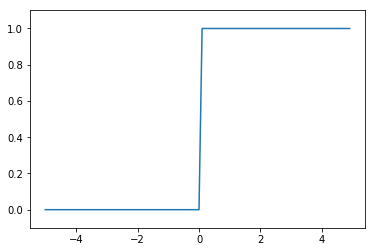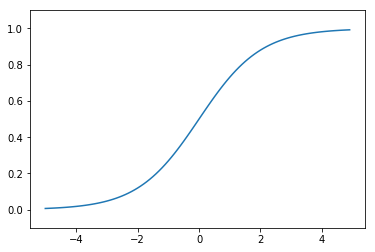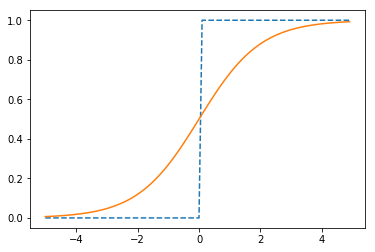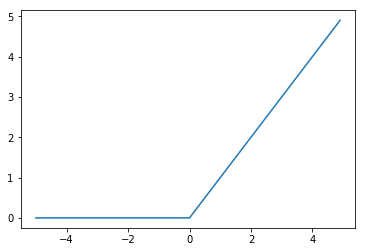# 深度学习之《深度学习入门》学习笔记（三）神经网络

2019/8/12 21:46 下午 posted in  随记

1. 即便对于复杂的函数，感知机也隐含着能够表示它的可能性。
2. 但设定权重的工作，即确定合适的、能符合预期的输入与输出的权重，现在还是由人工进行的。

## 激活函数

### sigmoid函数

$h(x)=\frac{1}{1+exp(-x)}$

import numpy as np
import matplotlib.pylab as plt
#实现简单的阶跃函数
def step_function0(x):
if x > 0:
return 1
else:
return 0

def step_function1(x):
y = x >0
return y.astype(np.int)

def step_function(x):
return np.array(x > 0, dtype=np.int)

x = np.arange(-5.0, 5.0, 0.1)
y = step_function(x)
plt.plot(x, y)
plt.ylim(-0.1, 1.1) # 指定y轴的范围
plt.show()#sigmoid函数
def sigmoid(x):
return 1 / (1 + np.exp(-x))

yy = sigmoid(x)
plt.plot(x,yy)
plt.ylim(-0.1,1.1)
plt.show()plt.plot(x,y,linestyle = "--",label="step")
plt.plot(x,yy,label="sigmoid")
plt.show()1. sigmoid函数具有平滑性。
2. 两个函数结构均是“输入小时，输出接近0或为0，随着输入增大，输出趋向于1或变为1”，也就是说，当输入信号为重要信息时，阶跃函数和sigmoid函数都会输出较大的值；当输入信号为不重要的信息时，两者都输出较小的值。
3. 不管输入信号多大多小，输出信号值范围在0-1之间。
4. 两者均为非线性函数。

### ReLU函数

ReLU函数是一个非常简单的函数。ReLU函数在输入大于0时，直接输出该值；在输入小于0时，输出0。ReLU函数可以表示为下面的式子：

$h(x)=\begin{cases} x&(x>0) \\ 0&(x\leq 0) \end{cases}$

#ReLU函数实现
def relu(x):
return np.maximum(0,x)

y3 = relu(x)
plt.plot(x,y3)
plt.show()## 多维数组的运算

# 一维数组
import numpy as np
A = np.array([1, 2, 3, 4])
print(A)


[1 2 3 4]

np.ndim(A)#获取数组维数


1

A.shape#获取数组的形状


(4,)

A.shape#其结果是个元组（tuple）


4

# 二维数组,也是矩阵
B = np.array([[1,2], [3,4], [5,6]])
print(B)


[[1 2]
[3 4]
[5 6]]

np.ndim(B)


2

B.shape


(3, 2)

# 矩阵乘法
C = np.array([[1,2],[3,4]])
C.shape


(2, 2)

D = np.array([[5,6],[7,8]])
D.shape


(2, 2)

np.dot(C,D)#乘积为点积


array([[19, 22],
[43, 50]])

np.dot(D,C)#绝大部分矩阵不满足乘法交换律


array([[23, 34],
[31, 46]])

### 神经网络的内积

# 使用NumPy矩阵实现神经网络
X=np.array([1,2])
X.shape


(2,)

W=np.array([[1,3,5],[2,4,6]])
print(W)


[[1 3 5]
[2 4 6]]

W.shape


(2, 3)

YY=np.dot(X,W)
print(YY)


[ 5 11 17]

## 3层神经网络的实现

# 多维数组实现A(1)=XW(1)+B
# 输入层到第1层的信号传递
X=np.array([1.0, 0.5])
W1=np.array([[0.1,0.3,0.5],[0.2,0.4,0.6]])
B1=np.array([0.1,0.2,0.3])

print(W1.shape)
print(X.shape)
print(B1.shape)


(2, 3)
(2,)
(3,)

A1=np.dot(X,W1)+B1
#观察第1层中激活函数的计算过程,激活函数使用sigmoid
Z1=sigmoid(A1)
print(A1)
print(Z1)


[0.3 0.7 1.1]
[0.57444252 0.66818777 0.75026011]

#第1层到第2层的信号传递
W2=np.array([[0.1,0.4],[0.2,0.5],[0.3,0.6]])
B2=np.array([0.1,0.2])

print(Z1.shape)
print(W2.shape)
print(B2.shape)
A2=np.dot(Z1,W2) + B2
Z2=sigmoid(A2)


(3,)
(3, 2)
(2,)

#第2层到输出层的信号传递
#定义输出层的激活函数为恒等函数
def identity_function(x):
return x

W3=np.array([[0.1,0.3],[0.2,0.4]])
B3=np.array([0.1,0.2])
A3=np.dot(Z2,W3)+B3
Y=identity_function(A3)
print(Y)


[0.31682708 0.69627909]

### 代码实现小结

# 3层网络实现案例，把权重记为大写字母W1，其他的偏置或中间结果等用小写字母表示
def init_network():
network = {}
network['W1'] = np.array([[0.1,0.3,0.5],[0.2,0.4,0.6]])
network['b1'] = np.array([0.1,0.2,0.3])
network['W2'] = np.array([[0.1,0.4],[0.2,0.5],[0.3,0.6]])
network['b2'] = np.array([0.1, 0.2])
network['W3'] = np.array([[0.1,0.3],[0.2,0.4]])
network['b3'] = np.array([0.1,0.2])

return network

def forward(network, x):
W1, W2, W3 = network['W1'], network['W2'], network['W3']
b1, b2, b3 = network['b1'], network['b2'], network['b3']

a1 = np.dot(x, W1) + b1
z1 = sigmoid(a1)
a2 = np.dot(z1, W2) + b2
z2 = sigmoid(a2)
a3 = np.dot(z2, W3) + b3
y = identity_function(a3)

return y

network = init_network()
x = np.array([1.0, 0.5])
y = forward(network, x)
print(y)


[0.31682708 0.69627909]

## 输出层的设计

### 恒等函数和softmax函数

$y_{k}=\frac{exp(a_{k})}{\sum_{i=1}^{n}exp(a_{i})}$

$$exp(x)=e^x$$。上式表示假设输出层共有$$m$$个神经元，计算第$$k$$个神经元的输出$$y_{k}$$。softmax函数的分子是输入信号$$a_{k}$$的指数函数，分母是所有输入信号的指数函数的和。

#实现softmax函数
a = np.array([0.3, 2.9, 4.0])
exp_a = np.exp(a) #指数函数
print(exp_a)
sum_exp_a = np.sum(exp_a) #指数函数的和
print(sum_exp_a)
y = exp_a / sum_exp_a
print(y)

[ 1.34985881 18.17414537 54.59815003]
74.1221542101633
[0.01821127 0.24519181 0.73659691]

# 定义softmax函数。供以后使用(初始版)
def softmax1(a):
exp_a = np.exp(a)
sum_exp_a = np.sum(exp_a)
y = exp_a / sum_exp_a

return y


### 实现softmax函数时的注意事项

softmax实现需要注意溢出问题，因为softmax函数内有$$exp(x)$$的指数运算，会使数值变得很大。因此，softmax函数的实现可以按如下式改进：

$y_{k}=\frac{exp(a_{k})}{\sum_{i=1}^{n}exp(a_{i})}=\frac{C exp(a_{k})}{C \sum_{i=1}^{n}exp(a_{i})}=\frac{exp(a_{k}+log C)}{\sum_{i=1}^{n}exp(a_{i}+log C)}=\frac{exp(a_{k}+C^{'})}{\sum_{i=1}^{n}exp(a_{i}+C^{'})}$

# 演示计算溢出情况与解决
a = np.array([1010, 1000, 990])
np.exp(a) / np.sum(np.exp(a)) #softmax,并未正确被计算，报错


/opt/conda/lib/python3.6/site-packages/ipykernel_launcher.py:3: RuntimeWarning: overflow encountered in exp
This is separate from the ipykernel package so we can avoid doing imports until
/opt/conda/lib/python3.6/site-packages/ipykernel_launcher.py:3: RuntimeWarning: invalid value encountered in true_divide
This is separate from the ipykernel package so we can avoid doing imports until

array([nan, nan, nan])

c = np.max(a) #1010
a - c
np.exp(a - c) / np.sum(np.exp(a - c))


array([9.99954600e-01, 4.53978686e-05, 2.06106005e-09])

# 定义softmax函数。供以后使用(正式用版)
def softmax(a):
c = np.max(a)
exp_a = np.exp(a - c)#溢出对策
sum_exp_a = np.sum(exp_a)
y = exp_a / sum_exp_a

return y


### softmax函数的特征

softmax函数的输出是0.0到1.0之间的实数。并且，softmax函数的输出值的总和是1.输出总和为1是softmax函数的一个重要特质，这个特质让softmax函数的输出解释为“概率”。通过softmax函数，我们可以用概率的（统计的）方法处理问题。

# 可解释为“概率”的softmax函数
a = np.array([0.3, 2.9, 4.0])
y = softmax(a)
print(y)
np.sum(y)


[0.01821127 0.24519181 0.73659691]

1.0

## 手写数字识别

# 代码暂略
import sys, os
print(sys.path.append(os.pardir))


None